CBSE Class 10 Sample Paper for 2018 Boards

Class 10
Solutions of Sample Papers for Class 10 Boards

### Two dairy owners A and B sell flavoured milk filled to capacity in mugs of negligible thickness, which are cylindrical in shape with a raised hemispherical bottom. The mugs are 14 cm high and have diameter of 7 cm as shown in given figure. Both A and B sell flavoured milk at the rate of  80 per litre. The dairy owner A uses the formula  𝜋 r 2 h to find the volume of milk in the mug and charges  43.12 for it. The dairy owner B is of the view that the price of actual quantity of milk should be charged. What according to him should be the price of one mug of milk? Which value is exhibited by the dairy owner B? (use π = 22/7)

This is a question of CBSE Sample Paper - Class 10 - 2017/18.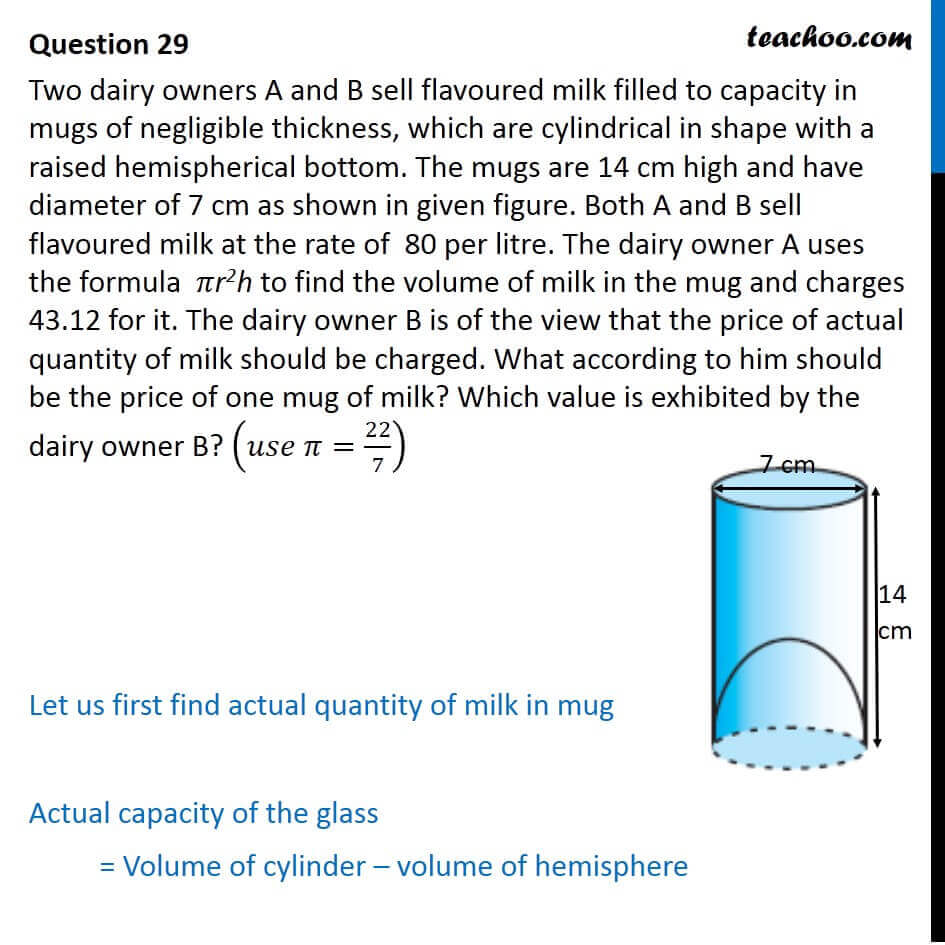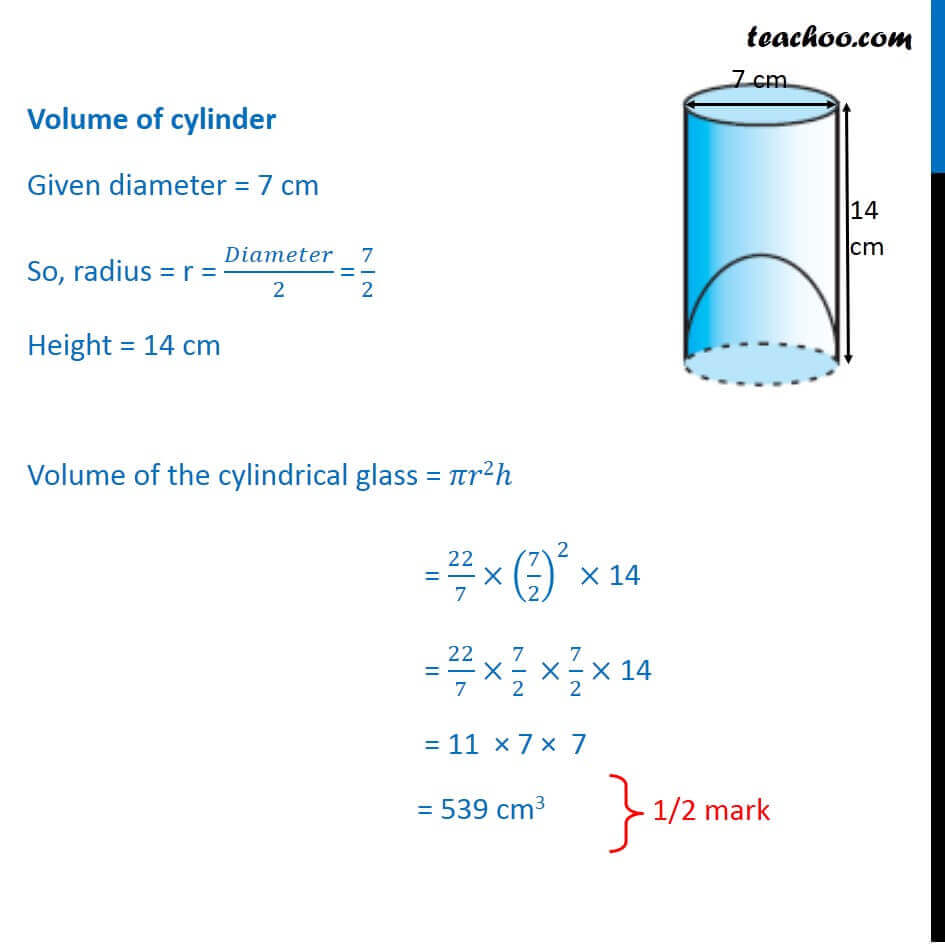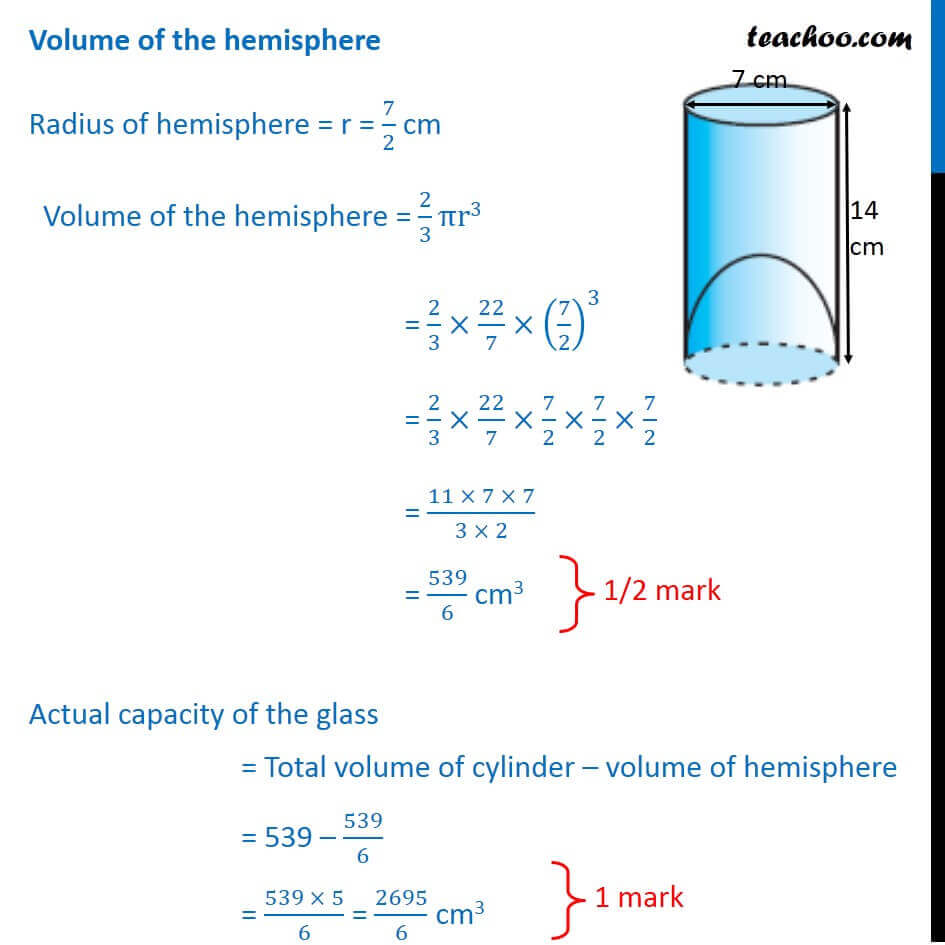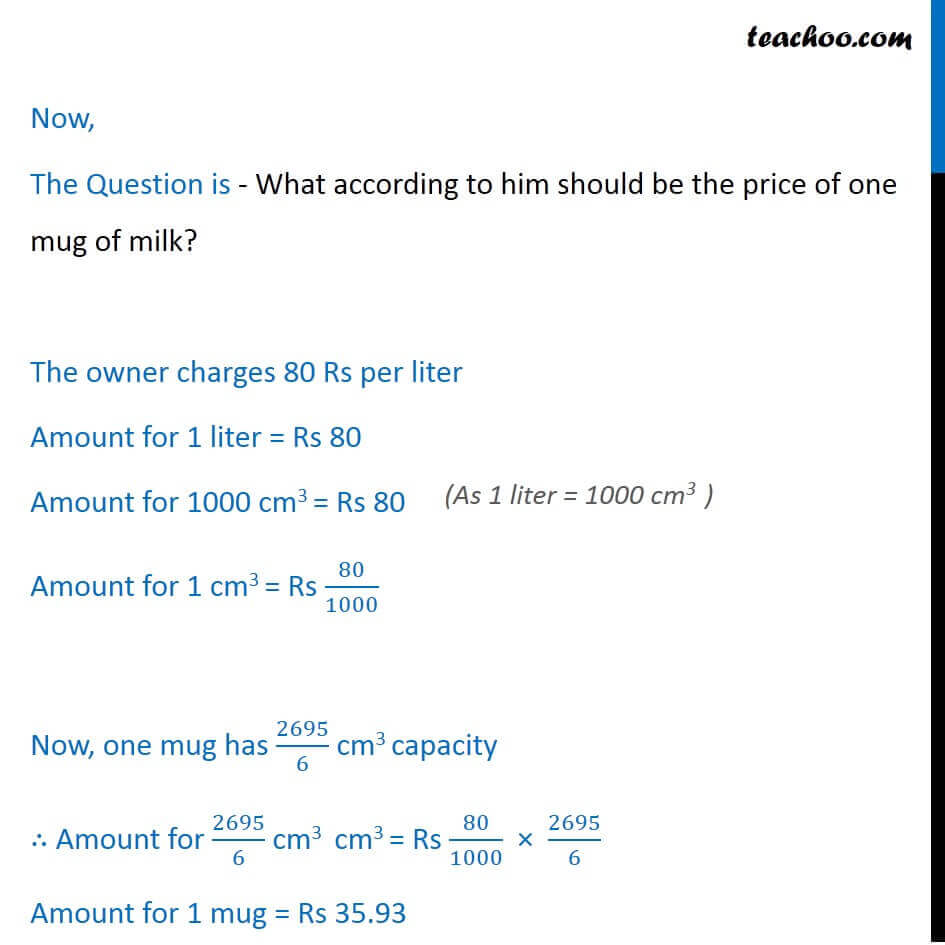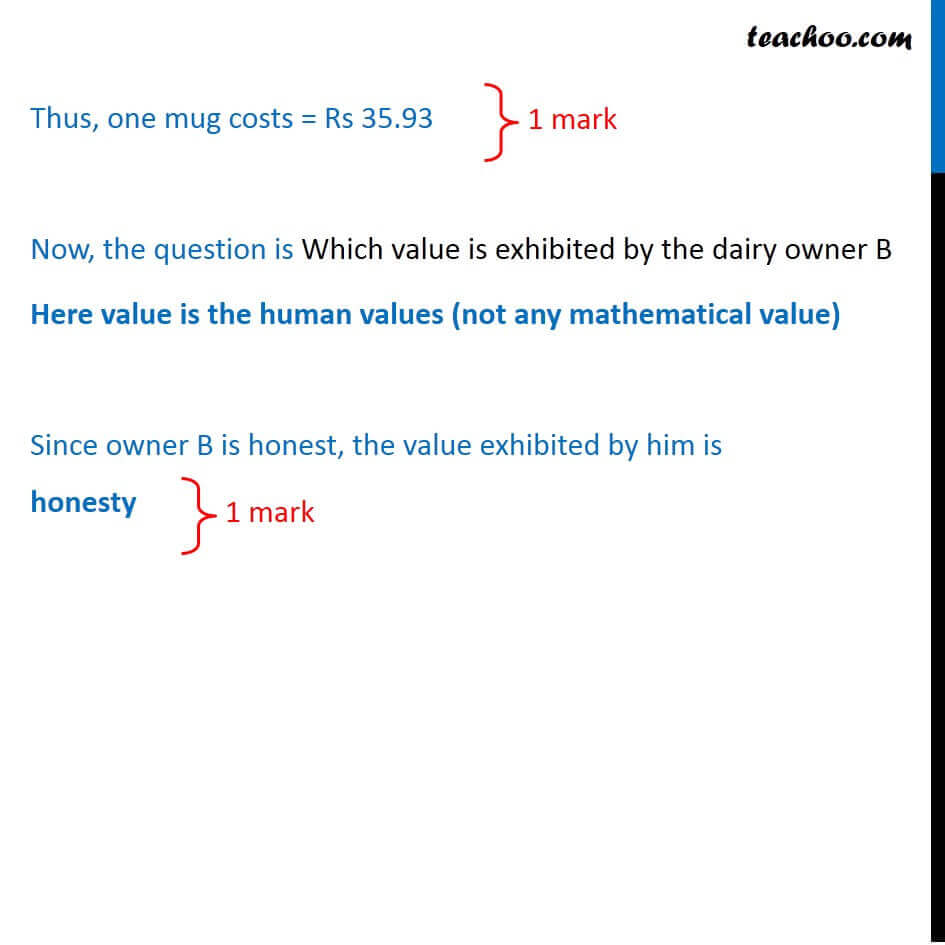Learn in your speed, with individual attention - Teachoo Maths 1-on-1 Class

### Transcript

Question 29 Two dairy owners A and B sell flavoured milk filled to capacity in mugs of negligible thickness, which are cylindrical in shape with a raised hemispherical bottom. The mugs are 14 cm high and have diameter of 7 cm as shown in given figure. Both A and B sell flavoured milk at the rate of 80 per litre. The dairy owner A uses the formula 𝜋r2h to find the volume of milk in the mug and charges 43.12 for it. The dairy owner B is of the view that the price of actual quantity of milk should be charged. What according to him should be the price of one mug of milk? Which value is exhibited by the dairy owner B? (𝑢𝑠𝑒 𝜋=22/7) Let us first find actual quantity of milk in mug Actual capacity of the glass = Volume of cylinder – volume of hemisphere Volume of cylinder Given diameter = 7 cm So, radius = r = 𝐷𝑖𝑎𝑚𝑒𝑡𝑒𝑟/2 "=" 7/2 Height = 14 cm Volume of the cylindrical glass = 𝜋𝑟2ℎ = 22/7 × (7/2)^2 × 14 = 22/7 ×7/2 ×7/2× 14 = 11 × 7 × 7 = 539 cm3 Volume of the hemisphere Radius of hemisphere = r = 7/2 cm Volume of the hemisphere = 2/3 πr3 = 2/3×22/7×(7/2)^3 = 2/3×22/7×7/2×7/2×7/2 = (11 × 7 × 7)/(3 × 2) = 539/6 cm3 Actual capacity of the glass = Total volume of cylinder – volume of hemisphere = 539 – 539/6 = (539 × 5)/6 = 2695/6 cm3 Now, The Question is - What according to him should be the price of one mug of milk? The owner charges 80 Rs per liter Amount for 1 liter = Rs 80 Amount for 1000 cm3 = Rs 80 Amount for 1 cm3 = Rs 80/1000 Now, one mug has 2695/6 cm3 capacity ∴ Amount for 2695/6 cm3 cm3 = Rs 80/1000 × 2695/6 Amount for 1 mug = Rs 35.93 Thus, one mug costs = Rs 35.93 Now, the question is Which value is exhibited by the dairy owner B Here value is the human values (not any mathematical value) Since owner B is honest, the value exhibited by him is honesty